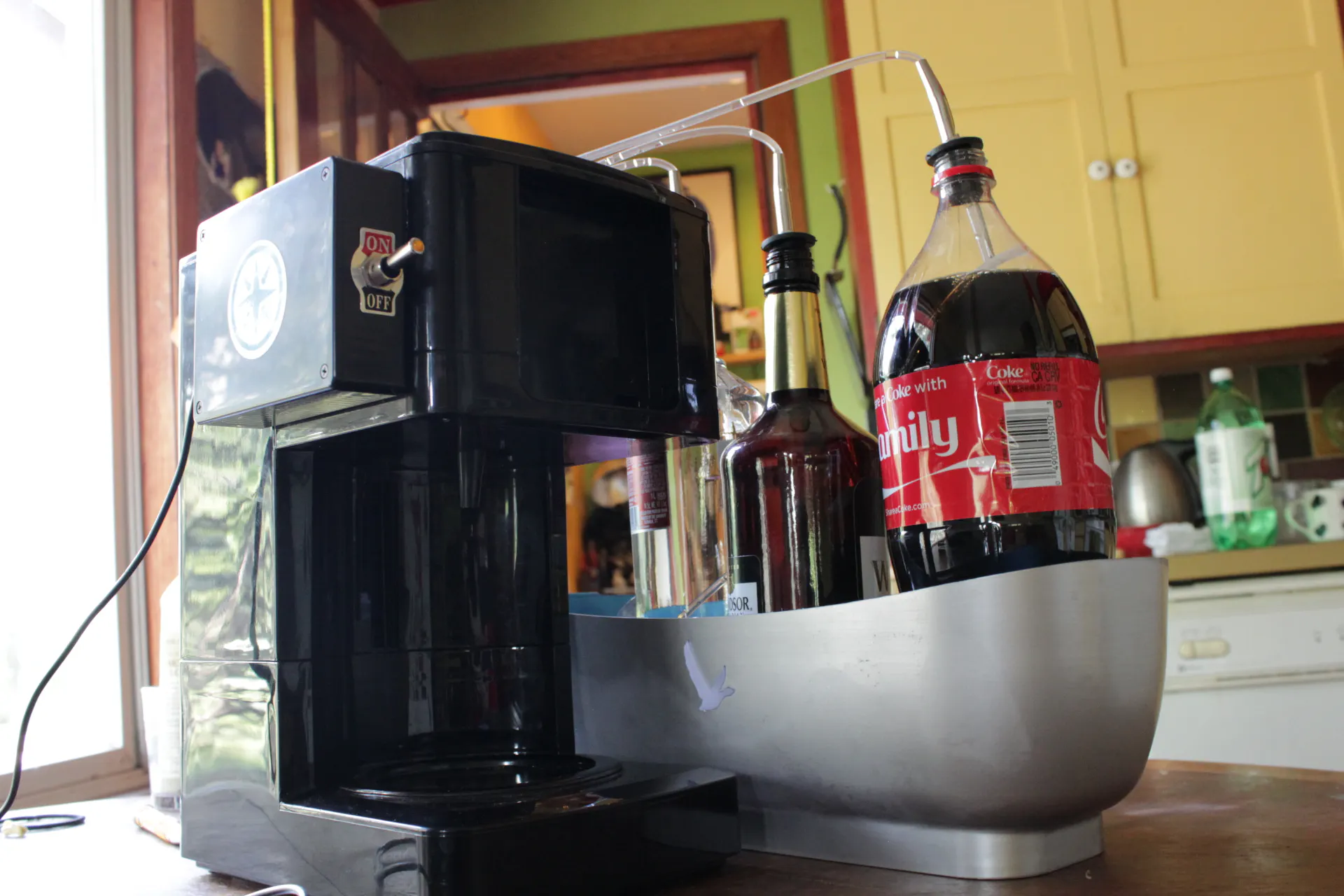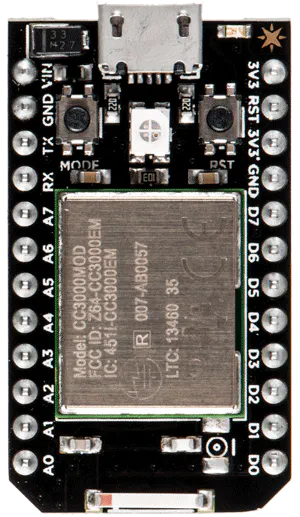Published

# The Foobar

A Spark-powered, automated, cocktail dispenser

Work in progress14,443## Things used in this project

### Hardware componentsParticle Spark Core
×1

## Code

### drinkVend.ino

C/C++
Firmware
```/*

drinkVend.ino is firmware for the foobar, a Spark-powered cocktail dispensery

*/

int myMotorArray; //initialize motor array so correct motor can be referenced
int toMaking = 0; //integer representation of whether there is a drink to be made
int sparkHandler(String command); //handler for the exposed Spark.function() that starts the ball rolling for the drink to be made.
String currCommand; //the current command of how long each pump should be turned on for the drink to be made. e.g. "12,12,0,6"
void strInter(String command); //helper function that transforms the string input "currCommand" of seconds to an array of integers
void pumpControl(); //function responsible for turning the correct pumps on/off, dispensing the drink.
unsigned long currentMillis; //current timestamp for pumpControl
unsigned long previousMillis; //last timestamp for pumpControl
unsigned long interval; //current interval for how long until the next pump is turned off
int motor1 = A0;
int motor2 = A1;
int motor3 = A4;
int motor4 = A5;

SYSTEM_MODE(MANUAL); //allows for control of Spark.connect() and Spark.process()

void setup() {

Serial.begin(9600);           // set up Serial library at 9600 bps

pinMode(motor1, OUTPUT);
pinMode(motor2, OUTPUT);
pinMode(motor3, OUTPUT);
pinMode(motor4, OUTPUT);

myMotorArray = motor1;
myMotorArray = motor2;
myMotorArray = motor3;
myMotorArray = motor4;

Spark.function("mix", sparkHandler);
Spark.variable("making", &toMaking, INT);

}

void loop() {

if (Spark.connected() == false){
Spark.connect();
}

else {

Spark.process();

if (toMaking){
strInter(currCommand);
toMaking = 0;
}

}

}

/*

sparkHandler is the handler function for a spark.function() "mix" exposed to the internet.
When the function is called, currCommand is set to the string passed into the function,
while toMaking is set to 1 so that the loop will trip, moving a step further towards a made drink by calling strInter().

*/

int sparkHandler(String command){

currCommand = command;
toMaking = 1;
return 1;

}

/*
strInter is a funtion called from the loop if toMaking has a value of 1. It takes in a string--currCommand--, which it will modify into an int array.
E.g. "12,12,0,6" will become an array of the same integers in the same order, [12,12,0,6]. Then the process is moved forward by calling drink()
on this array of integers.

Parameters: a string of four comma separated integers, intended to be placed in an array.

functions that call this function: loop()

drink-making baton is passed to: drink()
*/

void strInter(String instructions) {

int tymes;
char *pointer = strtok(strdup(instructions.c_str()), ",");

for(int i = 0; i < 4; i++){
tymes[i] = atoi(pointer);
Serial.println("motor " + String(i) + " : " + String(atoi(pointer)) + " seconds");
pointer = strtok(NULL, ",");
}

pumpControl(tymes);

}

/*
pumpControl is the function responsible for turning on/off the correct motors for the correct period of time. It does so by using the millis()
function for timing, using an additive cumulation of seconds to turn of the motors in the correct order.

Parameters: a length-4 integer array of the times of each motor to be turned on for.

functions that call this function: mix()

once this function call is complete, the drink making process should be complete, enjoy!

*/

void pumpControl(int tymes) {

int min = 10000;
int lastMin = 0;
int index = 0;
int running = 1;
int newMin = 1;
interval = 100000;
int count = 0;

previousMillis = millis();

digitalWrite(motor1, HIGH);
digitalWrite(motor2, HIGH);
digitalWrite(motor3, HIGH);
digitalWrite(motor4, HIGH);

while (running) {

if (newMin) {

for(int i = 0; i < 4; i++){ //find the next smallest pump time in the array

if ((tymes[i] < min) && (tymes[i] >= 0)) {
min = tymes[i];
Serial.println(String(i) + " min : " + String(min));
index = i;
}

}

interval = (min - lastMin) * 1000; //set interval to current smallest pump time - last smallest pump time (in ms)
tymes[index] = -1;
newMin = 0;
count++;
Serial.println(String(count) + " delay : " + String(interval));
}

currentMillis = millis();

if (currentMillis - previousMillis > interval) { //case where pump needs to be turned off

previousMillis = currentMillis;

digitalWrite(myMotorArray[index], LOW);

Serial.println("Motor "+String(index + 1) + " stop");
lastMin = min;
min = 10000;
newMin = 1;

}

if (count >= 4 && newMin == 1) {
running = 0;
Serial.println();
Serial.println("Done");
Serial.println();
}
}

return;
}
```

### Github

Github Repository

## Credits

### Max E.

5 projects • 23 followers
I like skateboarding, machines, and economics.